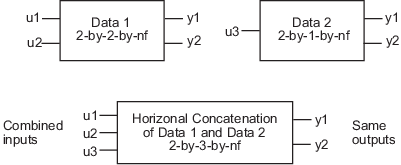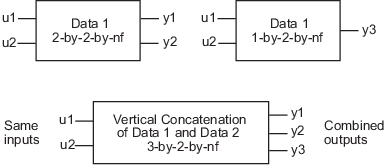Main Content

## Representing Frequency-Response Data Using idfrd Objects

### idfrd Constructor

The `idfrd` represents complex frequency-response data. Before you can create an `idfrd` object, you must import your data as described in Frequency-Response Data Representation.

Note

The `idfrd` object can only encapsulate one frequency-response data set. It does not support the `iddata` equivalent of multiexperiment data.

Use the following syntax to create the data object `fr_data`:

```fr_data = idfrd(response,f,Ts) ```

Suppose that `ny` is the number of output channels, `nu` is the number of input channels, and `nf` is a vector of frequency values. `response` is an `ny`-by-`nu`-by-`nf` 3-D array. `f` is the frequency vector that contains the frequencies of the response.`Ts` is the sample time, which is used when measuring or computing the frequency response. If you are working with a continuous-time system, set `Ts` to `0`.

`response(ky,ku,kf)`, where `ky`, `ku`, and `kf` reference the `k`th output, input, and frequency value, respectively, is interpreted as the complex-valued frequency response from input `ku` to output `ky` at frequency `f(kf)`.

Note

When you work at the command line, you can only create `idfrd` objects from complex values of G(eiw). For a SISO system, `response` can be a vector.

You can specify object properties when you create the `idfrd` object using the constructor syntax:

```fr_data = idfrd(response,f,Ts, 'Property1',Value1,...,'PropertyN',ValueN)```

### idfrd Properties

To view the properties of the `idfrd` object, you can use the `get` command. The following example shows how to create an `idfrd` object that contains 100 frequency-response values with a sample time of 0.1 s and get its properties:

```f = logspace(-1,1,100); [mag, phase] = bode(idtf([1 .2],[1 2 1 1]),f); response = mag.*exp(1j*phase*pi/180); fr_data = idfrd(response,f,0.1); get(fr_data)```
``` FrequencyUnit: 'rad/TimeUnit' Report: [1x1 idresults.frdest] SpectrumData: [] CovarianceData: [] NoiseCovariance: [] InterSample: {'zoh'} ResponseData: [1x1x100 double] IODelay: 0 InputDelay: 0 OutputDelay: 0 Ts: 0.1000 TimeUnit: 'seconds' InputName: {''} InputUnit: {''} InputGroup: [1x1 struct] OutputName: {''} OutputUnit: {''} OutputGroup: [1x1 struct] Notes: [0x1 string] UserData: [] Name: '' SamplingGrid: [1x1 struct] Frequency: [100x1 double] ```

For a complete description of all `idfrd` object properties, see the `idfrd` reference page.

To change property values for an existing `idfrd` object, use the `set` command or dot notation. For example, to change the name of the `idfrd` object, type the following command sequence at the prompt:

`fr_data.Name = 'DC_Converter';`

### Select I/O Channels and Data in idfrd Objects

You can reference specific data values in the `idfrd` object using the following syntax:

`fr_data(outputchannels,inputchannels)`

Reference specific channels by name or by channel index.

Tip

Use a colon (`:`) to specify all channels, and use the empty matrix (`[]`) to specify no channels.

For example, the following command references frequency-response data from input channel `3` to output channel `2`:

`fr_data(2,3) `

You can also access the data in specific channels using channel names. To list multiple channel names, use a cell array. For example, to retrieve the power output, and the voltage and speed inputs, use the following syntax:

`fr_data('power',{'voltage','speed'})`

To retrieve only the responses corresponding to frequency values between `200` and `300`, use the following command:

`fr_data_sub = fselect(fr_data,[200:300])`

You can also use logical expressions to subreference data. For example, to retrieve all frequency-response values between frequencies `1.27` and `9.3` in the `idfrd` object `fr_data`, use the following syntax:

`fr_data_sub = fselect(fr_data,fr_data.f>1.27&fr_data.f<9.3)`

Tip

Use `end` to reference the last sample number in the data. For example, `data(77:end)`.

Note

You do not need to type the entire property name. In this example, `f` in `fr_data.f` uniquely identifies the `Frequency` property of the `idfrd` object.

### Adding Input or Output Channels in idfrd Objects

#### About Concatenating idfrd Objects

The horizontal and vertical concatenation of `idfrd` objects combine information in the `ResponseData` properties of these objects. `ResponseData` is an `ny`-by-`nu`-by-`nf` array that stores the response of the system, where `ny` is the number of output channels, `nu` is the number of input channels, and `nf` is a vector of frequency values (see the `ResponseData` property description in `idfrd`).

#### Horizontal Concatenation of idfrd Objects

The following syntax creates a new `idfrd` object `data` that contains the horizontal concatenation of `data1,data2,...,dataN`:

`data = [data1,data2,...,dataN]`

`data` contains the frequency responses from all of the inputs in `data1,data2,...,dataN` to the same outputs. The following diagram is a graphical representation of horizontal concatenation of frequency-response data. The `(j,i,:)` vector of the resulting response data represents the frequency response from the `i`th input to the `j`th output at all frequencies.Note

Horizontal concatenation of `idfrd` objects requires that they have the same outputs and frequency vectors. If the output channel names are different and their dimensions are the same, the concatenation operation resets the output names to their default values.

#### Vertical Concatenation of idfrd Objects

The following syntax creates a new `idfrd` object `data` that contains the vertical concatenation of `data1,data2,...,dataN`:

`data = [data1;data2;... ;dataN]`

The resulting `idfrd` object `data` contains the frequency responses from the same inputs in `data1,data2,...,dataN` to all the outputs. The following diagram is a graphical representation of vertical concatenation of frequency-response data. The `(j,i,:)` vector of the resulting response data represents the frequency response from the `i`th input to the `j`th output at all frequencies.Note

Vertical concatenation of `idfrd` objects requires that they have the same inputs and frequency vectors. If the input channel names are different and their dimensions are the same, the concatenation operation resets the input names to their default values.

#### Concatenating Noise Spectrum Data of idfrd Objects

When the `SpectrumData` property of individual `idfrd` objects is not empty, horizontal and vertical concatenation handle `SpectrumData`, as follows.

In case of horizontal concatenation, there is no meaningful way to combine the `SpectrumData` of individual `idfrd` objects and the resulting `SpectrumData` property is empty. An empty property results because each `idfrd` object has its own set of noise channels, where the number of noise channels equals the number of outputs. When the resulting `idfrd` object contains the same output channels as each of the individual `idfrd` objects, it cannot accommodate the noise data from all the `idfrd` objects.

In case of vertical concatenation, the toolbox concatenates individual noise models diagonally. The following shows that `data.SpectrumData` is a block diagonal matrix of the power spectra and cross spectra of the output noise in the system:

`$data.s=\left(\begin{array}{ccc}data1.s& & 0\\ & \ddots & \\ 0& & dataN.s\end{array}\right)$`

`s` in `data.s` is the abbreviation for the `SpectrumData` property name.

### Operations that Create idfrd Objects

The following operations create `idfrd` objects:

• Constructing `idfrd` objects.

• Estimating nonparametric models using `etfe`, `spa`, and `spafdr`. For more information, see Frequency-Response Models.

• Converting the Control System Toolbox™ `frd` object. For more information, see Using Identified Models for Control Design Applications.

• Converting any linear dynamic system using the `idfrd` command.

For example:

```sys_idpoly = idpoly([1 2 1],[0 2],'Ts',1); G = idfrd(sys_idpoly,linspace(0,pi,128))```
```G = IDFRD model. Contains Frequency Response Data for 1 output(s) and 1 input(s), and the spectra for disturbances at the outputs. Response data and disturbance spectra are available at 128 frequency points, ranging from 0 rad/s to 3.142 rad/s. Sample time: 1 seconds Status: Created by direct construction or transformation. Not estimated. ```

## SupportGet trial now